Related Articles

# Implementation of Quick sort using MPI, OMP and Posix thread

• Last Updated : 22 Sep, 2021

QuickSort is a Divide and Conquer Algorithm. It picks an element as a pivot and partitions the array around the picked pivot. There are many ways of choosing the pivot elements. They are:

• Always pick the first element as a pivot.
• Always pick the last element as the pivot (implemented below)
• Pick a random element as a pivot.
• Pick median as a pivot.

MPI: MPI stands for Message Passing Interface. Here the message is data. MPI allows data to be passed between processes in a distributed memory environment. In C, “mpi.h” is a header file that includes all data structures, routines, and constants of MPI. Using “mpi.h” parallelized the quick sort algorithm.  Below is the C program to implement quicksort using MPI:

## C

 `// C program to implement the Quick Sort``// Algorithm using MPI``#include ``#include ``#include ``#include ``#include ``using` `namespace` `std;` `// Function to swap two numbers``void` `swap(``int``* arr, ``int` `i, ``int` `j)``{``    ``int` `t = arr[i];``    ``arr[i] = arr[j];``    ``arr[j] = t;``}` `// Function that performs the Quick Sort``// for an array arr[] starting from the``// index start and ending at index end``void` `quicksort(``int``* arr, ``int` `start, ``int` `end)``{``    ``int` `pivot, index;` `    ``// Base Case``    ``if` `(end <= 1)``        ``return``;` `    ``// Pick pivot and swap with first``    ``// element Pivot is middle element``    ``pivot = arr[start + end / 2];``    ``swap(arr, start, start + end / 2);` `    ``// Partitioning Steps``    ``index = start;` `    ``// Iterate over the range [start, end]``    ``for` `(``int` `i = start + 1; i < start + end; i++) {` `        ``// Swap if the element is less``        ``// than the pivot element``        ``if` `(arr[i] < pivot) {``            ``index++;``            ``swap(arr, i, index);``        ``}``    ``}` `    ``// Swap the pivot into place``    ``swap(arr, start, index);` `    ``// Recursive Call for sorting``    ``// of quick sort function``    ``quicksort(arr, start, index - start);``    ``quicksort(arr, index + 1, start + end - index - 1);``}` `// Function that merges the two arrays``int``* merge(``int``* arr1, ``int` `n1, ``int``* arr2, ``int` `n2)``{``    ``int``* result = (``int``*)``malloc``((n1 + n2) * ``sizeof``(``int``));``    ``int` `i = 0;``    ``int` `j = 0;``    ``int` `k;` `    ``for` `(k = 0; k < n1 + n2; k++) {``        ``if` `(i >= n1) {``            ``result[k] = arr2[j];``            ``j++;``        ``}``        ``else` `if` `(j >= n2) {``            ``result[k] = arr1[i];``            ``i++;``        ``}` `        ``// Indices in bounds as i < n1``        ``// && j < n2``        ``else` `if` `(arr1[i] < arr2[j]) {``            ``result[k] = arr1[i];``            ``i++;``        ``}` `        ``// v2[j] <= v1[i]``        ``else` `{``            ``result[k] = arr2[j];``            ``j++;``        ``}``    ``}``    ``return` `result;``}` `// Driver Code``int` `main(``int` `argc, ``char``* argv[])``{``    ``int` `number_of_elements;``    ``int``* data = NULL;``    ``int` `chunk_size, own_chunk_size;``    ``int``* chunk;``    ``FILE``* file = NULL;``    ``double` `time_taken;``    ``MPI_Status status;` `    ``if` `(argc != 3) {``        ``printf``(``"Desired number of arguments are not thier "``               ``"in argv....\n"``);``        ``printf``(``"2 files required first one input and "``               ``"second one output....\n"``);``        ``exit``(-1);``    ``}` `    ``int` `number_of_process, rank_of_process;``    ``int` `rc = MPI_Init(&argc, &argv);` `    ``if` `(rc != MPI_SUCCESS) {``        ``printf``(``"Error in creating MPI "``               ``"program.\n "``               ``"Terminating......\n"``);``        ``MPI_Abort(MPI_COMM_WORLD, rc);``    ``}` `    ``MPI_Comm_size(MPI_COMM_WORLD, &number_of_process);``    ``MPI_Comm_rank(MPI_COMM_WORLD, &rank_of_process);` `    ``if` `(rank_of_process == 0) {``        ``// Opening the file``        ``file = ``fopen``(argv, ``"r"``);` `        ``// Printing Error message if any``        ``if` `(file == NULL) {``            ``printf``(``"Error in opening file\n"``);``            ``exit``(-1);``        ``}` `        ``// Reading number of Elements in file ...``        ``// First Value in file is number of Elements``        ``printf``(``            ``"Reading number of Elements From file ....\n"``);``        ``fscanf``(file, ``"%d"``, &number_of_elements);``        ``printf``(``"Number of Elements in the file is %d \n"``,``               ``number_of_elements);` `        ``// Computing chunk size``    ``chunk_size = (number_of_elements %``                  ``number_of_process == 0) ?``                  ``(number_of_elements /``                   ``number_of_process) :``                  ``(number_of_elements /``                  ``(number_of_process - 1);` `    ``data = (``int` `*)``malloc``(number_of_process *``                         ``chunk_size *``                         ``sizeof``(``int``));``    ` `    ``// Reading the rest elements in which``    ``// operation is being performed``    ``printf``(``"Reading the array from the file.......\n"``);``    ``for``(``int` `i = 0; i < number_of_elements; i++)``    ``{``            ``fscanf``(file, ``"%d"``, &data[i]);``    ``}` `    ``// Padding data with zero``    ``for``(``int` `i = number_of_elements;``            ``i < number_of_process *``                ``chunk_size; i++)``    ``{``            ``data[i] = 0;``    ``}` `    ``// Printing the array read from file``      ``printf``(``"Elements in the array is : \n"``);``      ``for``(``int` `i = 0; i < number_of_elements; i++)``      ``{``            ``printf``(``"%d  "``, data[i]);``      ``}` `      ``printf``(``"\n"``);` `      ``fclose``(file);``      ``file = NULL;``    ``}` `    ``// Blocks all process until reach this point``    ``MPI_Barrier(MPI_COMM_WORLD);` `    ``// Starts Timer``    ``time_taken -= MPI_Wtime();` `    ``// BroadCast the Size to all the``    ``// process from root process``    ``MPI_Bcast(&number_of_elements, 1, MPI_INT, 0,``              ``MPI_COMM_WORLD);` `    ``// Computing chunk size``  ``chunk_size= (number_of_elements %``               ``number_of_process == 0) ?``              ``(number_of_elements /``               ``number_of_process) :``              ``(number_of_elements /``              ``(number_of_process - 1);` `  ``// Calculating total size of chunk``  ``// according to bits``  ``chunk = (``int` `*)``malloc``(chunk_size *``                        ``sizeof``(``int``));` `  ``// Scatter the chuck size data to all process``  ``MPI_Scatter(data, chunk_size, MPI_INT, chunk,``              ``chunk_size, MPI_INT, 0, MPI_COMM_WORLD);``  ``free``(data);``  ``data = NULL;` `  ``// Compute size of own chunk and``               ``// then sort them``  ``// using quick sort` `  ``own_chunk_size = (number_of_elements >=``                    ``chunk_size*(rank_of_process + 1)) ?``                    ``chunk_size : (number_of_elements -``                                  ``chunk_size*rank_of_process);` `  ``// Sorting array with quick sort for every``  ``// chunk as called by process``  ``quicksort(chunk, 0, own_chunk_size);` `  ``for``(``int` `step = 1; step < number_of_process; step = 2 * step)``  ``{``        ``if` `(rank_of_process % (2 * step) != 0) {``            ``MPI_Send(chunk, own_chunk_size, MPI_INT,``                     ``rank_of_process - step, 0,``                     ``MPI_COMM_WORLD);``            ``break``;``        ``}` `        ``if` `(rank_of_process + step < number_of_process) {``            ``int` `received_chunk_size``                ``= (number_of_elements``                   ``>= chunk_size``                          ``* (rank_of_process + 2 * step))``                      ``? (chunk_size * step)``                      ``: (number_of_elements``                         ``- chunk_size``                               ``* (rank_of_process + step));``            ``int``* chunk_received;``            ``chunk_received = (``int``*)``malloc``(``                ``received_chunk_size * ``sizeof``(``int``));``            ``MPI_Recv(chunk_received, received_chunk_size,``                     ``MPI_INT, rank_of_process + step, 0,``                     ``MPI_COMM_WORLD, &status);` `            ``data = merge(chunk, own_chunk_size,``                         ``chunk_received,``                         ``received_chunk_size);` `            ``free``(chunk);``            ``free``(chunk_received);``            ``chunk = data;``            ``own_chunk_size``                ``= own_chunk_size + received_chunk_size;``        ``}``  ``}` `  ``// Stop the timer``  ``time_taken += MPI_Wtime();` `  ``// Opening the other file as taken form input``  ``// and writing it to the file and giving it``  ``// as the output``  ``if``(rank_of_process == 0)``  ``{``        ``// Opening the file``        ``file = ``fopen``(argv, ``"w"``);` `        ``if` `(file == NULL) {``            ``printf``(``"Error in opening file... \n"``);``            ``exit``(-1);``        ``}` `        ``// Printing total number of elements``        ``// in the file``        ``fprintf``(``            ``file,``            ``"Total number of Elements in the array : %d\n"``,``            ``own_chunk_size);` `        ``// Printing the value of array in the file``        ``for` `(``int` `i = 0; i < own_chunk_size; i++) {``            ``fprintf``(file, ``"%d  "``, chunk[i]);``        ``}` `        ``// Closing the file``        ``fclose``(file);` `        ``printf``(``"\n\n\n\nResult printed in output.txt file "``               ``"and shown below: \n"``);` `        ``// For Printing in the terminal``        ``printf``(``"Total number of Elements given as input : "``               ``"%d\n"``,``               ``number_of_elements);``        ``printf``(``"Sorted array is: \n"``);` `        ``for` `(``int` `i = 0; i < number_of_elements; i++) {``            ``printf``(``"%d  "``, chunk[i]);``        ``}` `        ``printf``(``            ``"\n\nQuicksort %d ints on %d procs: %f secs\n"``,``            ``number_of_elements, number_of_process,``            ``time_taken);``  ``}` `  ``MPI_Finalize();``  ``return` `0;``}`

Output: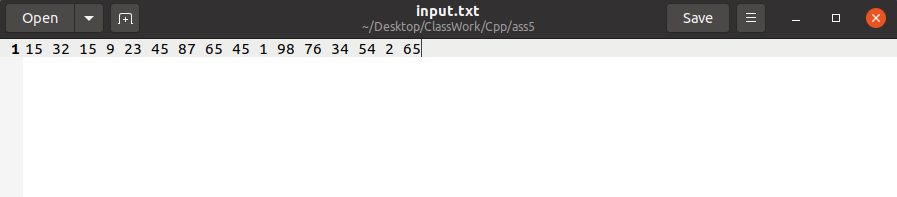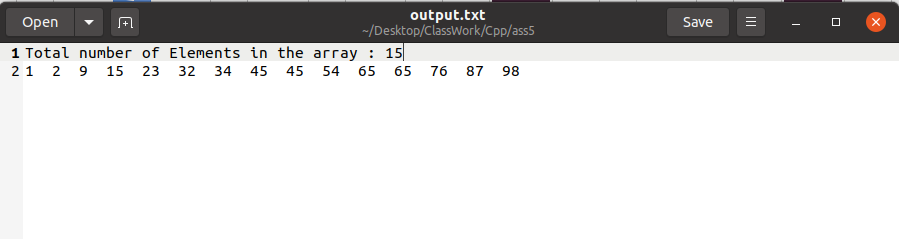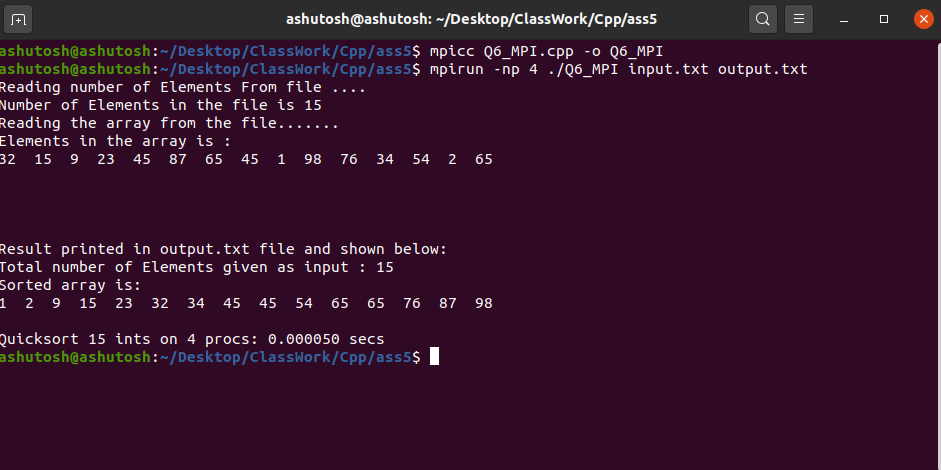OMP: OMP is Open Multi-Processing. It’s an Application Program Interface (API) that may be used to explicitly direct multi-threaded, shared memory parallelism. In C/ C++, “omp.h” is a header file that includes all the related directives related to OMP. Using “omp.h” parallelized quick sort. Below is the C++ program to implement the above concept:

## C++

 `// C++ program to implement the Quick Sort``// using OMI``#include ``#include ``using` `namespace` `std;` `// Function to swap two numbers a and b``void` `swap(``int``* a, ``int``* b)``{``    ``int` `t = *a;``    ``*a = *b;``    ``*b = t;``}` `// Function to perform the partitioning``// of array arr[]``int` `partition(``int` `arr[], ``int` `start, ``int` `end)``{``    ``// Declaration``    ``int` `pivot = arr[end];``    ``int` `i = (start - 1);` `    ``// Rearranging the array``    ``for` `(``int` `j = start; j <= end - 1; j++) {``        ``if` `(arr[j] < pivot) {``            ``i++;``            ``swap(&arr[i], &arr[j]);``        ``}``    ``}``    ``swap(&arr[i + 1], &arr[end]);` `    ``// Returning the respective index``    ``return` `(i + 1);``}` `// Function to perform QuickSort Algorithm``// using openmp``void` `quicksort(``int` `arr[], ``int` `start, ``int` `end)``{``    ``// Declaration``    ``int` `index;` `    ``if` `(start < end) {` `        ``// Getting the index of pivot``        ``// by partitioning``        ``index = partition(arr, start, end);` `// Parallel sections``#pragma omp parallel sections``        ``{``#pragma omp section``            ``{``                ``// Evaluating the left half``                ``quicksort(arr, start, index - 1);``            ``}``#pragma omp section``            ``{``                ``// Evaluating the right half``                ``quicksort(arr, index + 1, end);``            ``}``        ``}``    ``}``}` `// Driver Code``int` `main()``{``    ``// Declaration``    ``int` `N;` `    ``// Taking input the number of``    ``// elements we wants``    ``cout << ``"Enter the number of elements"``         ``<< ``" you want to Enter\n"``;``    ``cin >> N;` `    ``// Declaration of array``    ``int` `arr[N];` `    ``cout << ``"Enter the array: \n"``;` `    ``// Taking input that array``    ``for` `(``int` `i = 0; i < N; i++) {``        ``cin >> arr[i];``    ``}` `    ``// Calling quicksort having parallel``    ``// code implementation``    ``quicksort(arr, 0, N - 1);` `    ``// Printing the sorted array``    ``cout << ``"Array after Sorting is: \n"``;` `    ``for` `(``int` `i = 0; i < N; i++) {``        ``cout << arr[i] << ``" "``;``    ``}` `    ``return` `0;``}`

Output: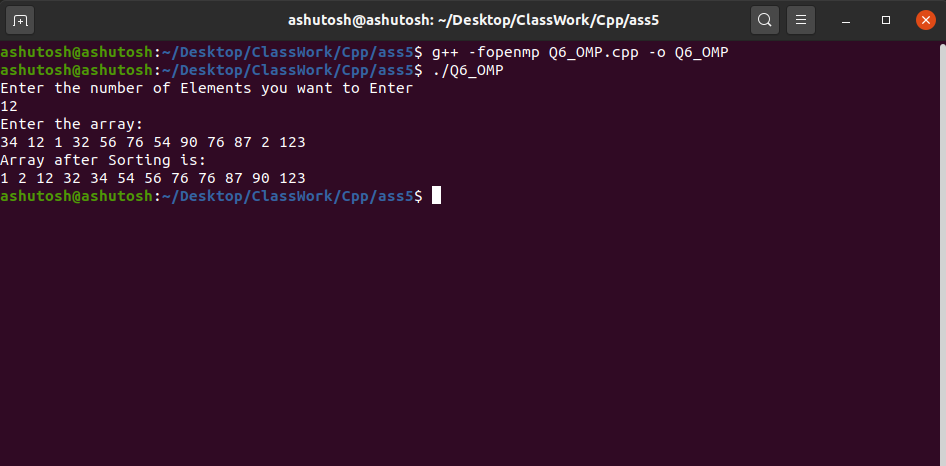POSIX Threads: The POSIX thread libraries are a C/C++ thread API based on standards. It enables the creation of a new concurrent process flow. It works well on multi-processor or multi-core systems, where the process flow may be scheduled to execute on another processor, increasing speed through parallel or distributed processing. Below is the C++ program to implement quicksort using POSIX threads:

## C++14

 `// C++ program to implement the Quick Sort``// using POSIX Thread``#include ``#include ``using` `namespace` `std;` `// Structure``struct` `data_set {``    ``int` `start_index;``    ``int` `end_index;``    ``int``* data;``};` `// Function to perform swap operations``void` `swap(``int``* a, ``int``* b)``{``    ``int` `t = *a;``    ``*a = *b;``    ``*b = t;``}` `// Partition function for making``// partition in array``int` `partition(``int` `arr[], ``int` `left_index,``              ``int` `right_index)``{``    ``// Declaration and initialization``    ``// choosing pivot element form which``    ``// we make partition` `    ``// Here pivot is last element of``    ``// the array``    ``int` `pivot = arr[right_index];``    ``int` `i = left_index - 1;` `    ``// Making array as per requirement``    ``// arranging element smaller than``    ``// pivot on left side and larger``    ``// then pivot on right side``    ``for` `(``int` `j = left_index;``         ``j <= right_index - 1; j++) {` `        ``if` `(arr[j] < pivot) {``            ``i++;``            ``swap(&arr[i], &arr[j]);``        ``}``    ``}` `    ``swap(&arr[i + 1], &arr[right_index]);` `    ``// Returning the partition index``    ``return` `i + 1;``}` `// Quicksort Function for sorting``// array``void``* quick_sort(``void``* data)``{``    ``// Retrieving back the data sent``    ``// from thread``    ``struct` `data_set* info = (``struct` `data_set*)data;` `    ``// Declaration of left index``    ``int` `left_index, right_index, index;` `    ``// Initialization of left and``    ``// right index``    ``left_index = info->start_index;``    ``right_index = info->end_index;` `    ``// Recursive call of quick_sort``    ``// function``    ``if` `(left_index < right_index) {` `        ``// Declaration of pthread and``        ``// pthread attribute type object``        ``pthread_attr_t attr;``        ``pthread_t first_thread;``        ``pthread_t second_thread;` `        ``// Making two pointers of type``        ``// data_set for making again``        ``// call form thread``        ``struct` `data_set* info1 = ``new` `data_set;``        ``struct` `data_set* info2 = ``new` `data_set;` `        ``// Their initialization``        ``info1->data = info->data;``        ``info2->data = info->data;` `        ``// Initialize of pthread attribute``        ``pthread_attr_init(&attr);` `        ``// For setting the set detach``        ``// state of attribute``        ``pthread_attr_setdetachstate(``            ``&attr, PTHREAD_CREATE_JOINABLE);` `        ``// Partition the array for any``        ``// recursive call``        ``index = partition(info->data,``                          ``left_index,``                          ``right_index);` `        ``info1->start_index = left_index;``        ``info1->end_index = index - 1;` `        ``// Create pthread type object and``        ``// printing the error if any``        ``if` `(pthread_create(&first_thread,``                           ``&attr, quick_sort,``                           ``info1)) {``            ``cout << ``"Error in creating thread "``                 ``<< endl;` `            ``// Exiting in case of not``            ``// creation of thread``            ``exit``(-1);``        ``}` `        ``info2->start_index = index + 1;``        ``info2->end_index = right_index;` `        ``// Creating pthread type object``        ``// and print the error``        ``if` `(pthread_create(&second_thread,``                           ``&attr, quick_sort,``                           ``info2)) {``            ``cout << ``"Error in creating thread "``                 ``<< endl;` `            ``// Exiting in case of not``            ``// creation of thread``            ``exit``(-1);``        ``}` `        ``// Joining the threads``        ``pthread_join(first_thread, NULL);``        ``pthread_join(second_thread, NULL);``    ``}` `    ``return` `NULL;``}` `// Driver Code``int` `main()``{``    ``// Declaration of Number of threads``    ``int` `N;` `    ``struct` `data_set* info = ``new` `data_set;` `    ``// Taking number of elements as input``    ``cout << ``"Enter number of elements"``         ``<< ``" in the array: \n"``;``    ``cin >> N;` `    ``// Declaration of array``    ``int` `A[N];` `    ``// Initialization of array``    ``cout << ``"Enter the array: "` `<< endl;``    ``for` `(``int` `i = 0; i < N; i++) {``        ``cin >> A[i];``    ``}` `    ``// Initialize of structure of``    ``// data_set type``    ``info->data = A;``    ``info->start_index = 0;``    ``info->end_index = N - 1;` `    ``// Declaration of pthread object``    ``pthread_t thread_id;` `    ``// Creating and pthread object and``    ``// printing the array of any``    ``if` `(pthread_create(&thread_id, NULL,``                       ``quick_sort,``                       ``info)) {``        ``cout << ``"Error in creating thread"``             ``<< endl;` `        ``// Exit in case of error``        ``exit``(-1);``    ``}` `    ``// Joining the pthread object``    ``int` `r1 = pthread_join(thread_id, NULL);` `    ``// Printing the array if any in case``    ``// of joining``    ``if` `(r1) {``        ``cout << ``"Error in Joinging thread"``             ``<< endl;` `        ``// Exiting in case of error``        ``exit``(-1);``    ``}` `    ``// Printing the array after sorting``    ``cout << ``"Sorted Array is: "` `<< endl;` `    ``for` `(``int` `i = 0; i < N; i++) {``        ``cout << A[i] << ``" "``;``    ``}``    ``cout << endl;` `    ``// Exiting from pthread programming``    ``pthread_exit(NULL);` `    ``return` `0;``}`

Output: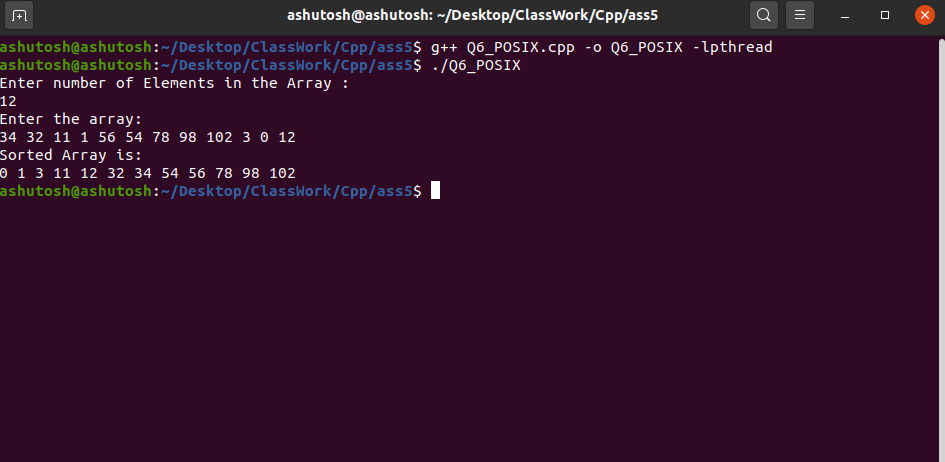Want to learn from the best curated videos and practice problems, check out the C++ Foundation Course for Basic to Advanced C++ and C++ STL Course for the language and STL. To complete your preparation from learning a language to DS Algo and many more, please refer Complete Interview Preparation Course.

My Personal Notes arrow_drop_up